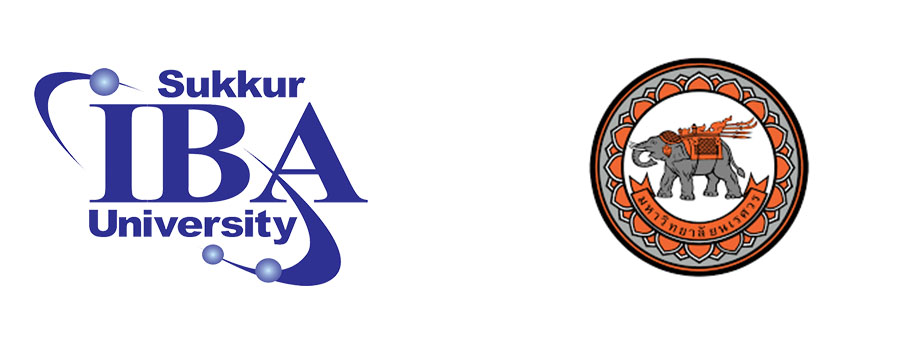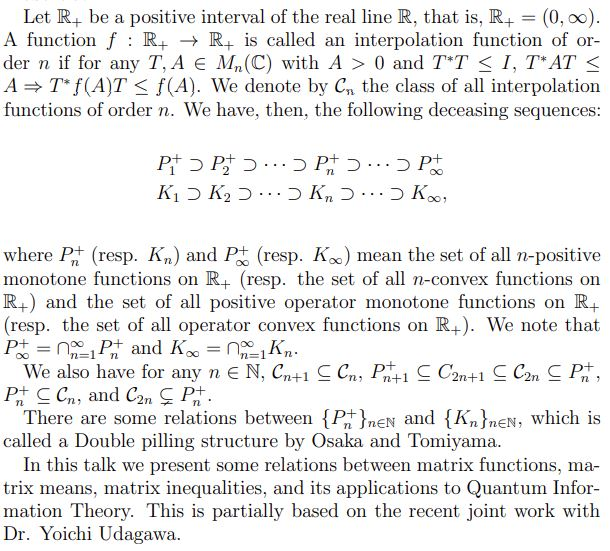### First SIBAU-NU Workshop on Matrix Analysis and Linear Algebra## Aims and Objectives

This is the first international online workshop on matrix analysis and its applications, hosted by the Department of Mathematics, Sukkur IBA University, (SIBAU)-Pakistan and Naresuan University, (NU)-Thailand.

This workshop will be held virtually during the period October 15-17, 2021. The theme of the workshop shall focus on a series of lectures on an introduction and recent development to matrix analysis and its applications.

The purpose of the workshop is to stimulate research and, in an informal setting, to foster the interaction of researchers working in applications of matrix theory. The workshop will provide a forum through which mathematicians may be better informed of the latest developments and newest techniques in linear algebra and matrix analysis and may exchange ideas with researchers from a wide variety of research fields from different parts of the world.

792 242 9795

5TD1UP

## Richard D. Braatz (MIT-USA)

Title of talk Applications of Matrix Theory to the Simulation, Analysis, and Optimal Control of Infinite-dimensional Singular Nonlinear Dynamical Systems

Abstract Many technological systems are described by nonlinear partial differential-algebraic equations (aka infinite-dimensional singular/descriptor nonlinear dynamical systems), which do not satisfy the assumptions made by the vast majority of methods for dynamical systems analysis and control design. Nearly all of this literature on this specific class of systems that there is a single spatially distributed state and that the system is linear time-invariant, which are assumptions that rarely hold for systems of technological importance such as in energy, chemical, material, and pharmaceutical processes. Another constraint relevant to the real-time control of these systems is the limitation on the computations available for carrying out real-time optimization-based control. This talk will describe some methods in which matrix theory has been applied in recent years to the simulation, analysis, and optimal control of systems of nonlinear partial differential-algebraic systems. These methods are shown to provide insights into the underlying physical phenomena, quantify the effects of parametric uncertainties, and generate control systems that are implementable in real time on standard control hardware. Methods are demonstrated for ethanol steam reforming which is described by more than a half dozen nonlinear partial differential algebraic equations. These results were in collaboration with Carlos Ocampo-Martinez and coworkers at the Universitat Politècnica de Catalunya.

## Hoa Dinh (Troy Uni-USA)

Title of talk Quantum divergences and some related questions

Abstract We introduce some new quantum divergences on the set of positive definite matrices in the relation with operator means. We solve the least squares problems with respect to new quantum divergence. In addition, we also study some geometric properties for operator means such as the in-betweenness property, the in-sphere property, Data Processing Inequality in Quantum information.

## Nicola Guglielmi (GSSI-Italy)

Title of talk Approximating dominant eigenpairs of a matrix valued linear operator.

Abstract I will propose a method to approximate the rightmost eigenpair of certain matrix-valued linear operators, in a low-rank setting. First, I will introduce an ODE, whose solution allows to approximate the rightmost eigenpair of the linear operator. Under generic assumptions the solution of the ODE converges globally to its leading eigen-matrix. The analysis of the projected operator on a low-rank manifold is more subtle due to its nonlinearity. When A is self-adjoint the associated low-rank ODE converges (at least locally) to its rightmost eigen-matrix in the low-rank manifold, a property which appears to hold in the more general case. Two explicit numerical methods are proposed, the second being an adaptation of the projector splitting integrator proposed recently by Lubich and Oseledets. The numerical experiments suggest that the method is effective and competitive.
This is inspired by a joint project with Carmen Scalone (Univ. of L'Aquila) and Daniel Kressner (EPFL, Lausanne).

## Stefano Massei (TU/e, Netherlands)

Title of talk Divide-and-conquer methods for matrix functions and matrix equations based on low-rank updates

Abstract Matrix functions, like the matrix exponential or square root, and matrix equations, such as the Sylvester and Lyapunov equations, play important roles in many applications, including network analysis, dimensionality reduction of dynamical control systems, and the solution of partial differential equations. In this context, when the input matrix coefficients undergo low-rank changes it is often observed a numerically low-rank variation of the outcome. In this talk, we present and analyze algorithms, based on Krylov subspaces, for quickly updating the solution of a matrix equation and quantities related to matrix functions, e.g. the diagonal and the trace. Then, we explain how to use such algorithms to obtain divide-and-conquer approaches for problems with coefficients that feature hierarchical low-rank structures, such as HODLR, HSS, and banded matrices. Numerical experiments demonstrate the advantages of divide-and-conquer over existing approaches, in terms of computational time and memory consumption.

## Hiroyuki Osaka (Ritsumeikan University-Japan)

Title of talk Interpolation functions and inequalities

Abstract## Gilbert Strang (MIT-USA)

Title of talk The Incidence Matrix of a Graph: The Matrix Factorization A = C R : A New Way to Begin a Linear Algebra Course

Abstract Each edge in a graph produces a row of the incidence matrix. The -1 and 1 in that row tell which nodes are connected by the edge. Graphs are fundamental in applied mathematics and these matrices have important properties. We find their rank and null space and echelon form and column-row factorization A = CR and pseudoinverse --- beautiful examples in linear algebra.

## Tin-Yau Tam (University of Nevada, Reno-USA)

Title of talk Inequalities of geometric mean and spectral mean of positive definite matrices

Abstract We first review the metric geometric mean introduced by Pusz and Woronowicz and the spectral geometric mean introduced by Fiedler and Pt\'ak, originally for n-by-n positive definite matrices. Some recent inequalities including log majorization related to t-metric geometric mean and \$t\$-spectral geometric mean are then given. Their extensions in the context of symmetric space associated with a noncompact semi simple Lie group will be mentioned. This is a joint work with Elaine Gan of the University of Nevada, Reno, USA.

## Fuzhen Zhang (Nova Southeastern University-USA)

Title of talk Singular Value Inequalities of log-Majorization Type

Abstract As a central topic of linear algebra and matrix theory, eigenvalues and singular values carry important properties of a matrix. We present some upper bounds for the product of arbitrarily selected singular values of the sum of two matrices.